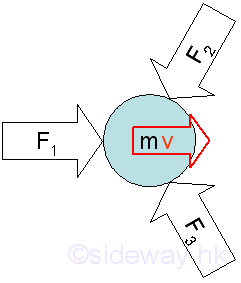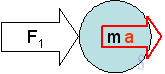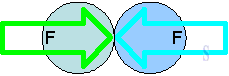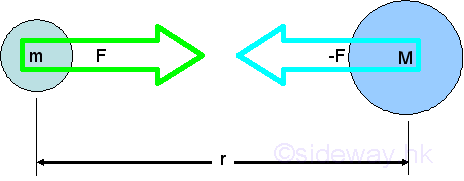output.to from Sideway
Mechanics

Draft for Information Only

## Introduction

Mechanics

Mechanics is a scientific study of objects under the action of forces by the description and prediction of their conditions in equilibrium or in motion.

The three areas of study are mechanics of rigid bodies, mechanics of deformable solids and mechanics of fluids.

For mechanics of rigid bodies, it can be subdivided into statics, and dynamics.

Principles

The study of mechanics are mainly based on the fundamental principles formulated by Newton in 1687.

Because of the implications of Einstein's first theory of relativity in 1905, the limitation of these principles are recognized. However, newtonian mechanics still applies in engineering sciences as the basis because the errors due to special relativity can be ignored at low speeds and weak gravitational fields.

The Principles includes Newton's three laws of motion, which are

• First Law: A particle continues its state at rest or in motion with constant speed in a straight line, when the resultant force acting on it is zero.• Second Law: When the resultant force acting on a particle is not zero, the net force will be equal to the time rate of change of the particle's linear momentum. As the mass is constant, the acceleration of the particle will be proportional to the magnitude and in the direction of the net applied force.• Third Law: For the forces of action on particles, there are always reaction forces which have the same magnitude along the same line of action but in opposite sense in response to the applied forces.And Newton's laws of gravitation, which states

• Every particle attracts other particle by a mutual force pointing to each other having same magnitude and opposite sense along the line intersecting both particles. The magnitude of the force is directly proportional to the product of the two particle masses and inversely proportional to the square of the distance between the particles.Concepts

The basic concepts used for the formulation of three laws of motion are space, time, mass, and force.

• Space is associated with the position of a point in a three dimensional system with reference to  a reference point, or an origin.

• Time is used to describe a succession of evevts

• Mass is used to characterize the inertia of an object by comparing the resistance of a material object to change in motion under exerting the necessary effort of action.

• Force is the effort used to change the motion of an object.

ID: 101200013 Last Updated: 1/14/2011 Revision: 1Home 5

Management

HBR 3

Information

Recreation

Culture

Chinese 1097

English 339

Computer

Hardware 226

Software

Application 213

Latex 52

Manim 205

KB 1

Numeric 19

Programming

Web 289

Unicode 504

HTML 66

CSS 65

SVG 46

ASP.NET 270

OS 429

Python 72

Knowledge

Mathematics

Algebra 84

Geometry 34

Calculus 67

Engineering

Mechanical

Mechanics 1

Rigid Bodies

Statics 92

Dynamics 37

Control

Natural Sciences

Electric 27